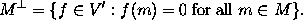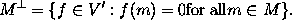Text Embedded in Displayed Equations

Text can be embedded in displayed equations (in LaTeX) by using \mbox{embedded text}. For example, one obtainsby typing
$M^\bot = \{ f \in V' : f(m) = 0 \mbox{ for all } m \in M \}.$
Note the blank spaces before and after the words `for all' in the above example. Had we typed
$M^\bot = \{ f \in V' : f(m) = 0 \mbox{for all} m \in M \}.$
we would have obtained